# Exception Handling in Python

## Exception Handling in Python with Examples

In this article, I am going to discuss Exception Handling in Python with examples. This is one of the most important concepts in Python. As a developer, while developing an application, it is your key responsibility to handle the exception. As part of this article, we are going to discuss the following pointers in detail.

1. Types of Error
2. Syntax and Runtime Errors
3. Normal and Abnormal Flow of Program Execution
4. What is an Exception?
5. Exception Handling in Python
6. Default Exception Handing in Python
7. Exception Hierarchy
8. How to Handle Exception in Python with Examples?
9. Printing Exception Information in Python
10. try with multiple except Blocks
11. One except block – Multiple Exceptions
12. Default Except Block in Python
##### Types of Error:

In any programming language, there are 2 types of errors possible. They are:

1. Syntax Errors
2. Runtime Errors
##### Syntax Errors

The errors which occur because of invalid syntax are called syntax errors.

###### Program: Syntax Error (demo1.py)
```x=123
if x==123
print("Hello")```

Output: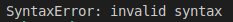Here we missed placing a colon in the if condition, which is violating the syntax. Hence, syntax error.

###### Program: Syntax Error (demo2.py)

print(‘hello’

Output: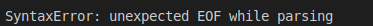The programmer is responsible to correct these syntax errors. Once all syntax errors are corrected then only program execution will be started.

##### Runtime Errors in Python

While executing the program if something goes wrong then we will get Runtime Errors. They might be caused due to,

1. End-User Input
2. Programming Logic
3. Memory Problems etc.

Such types of errors are called exceptions.

###### Program: Runtime Error (demo3.py)

print(10/0)

Output: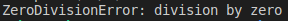###### Program: Runtime Error (demo4.py)

print(10/”two”)

Output: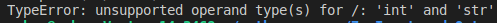###### Program: Runtime Error (demo4.py)
```x=int(input("Enter Number:"))
print(x)```
###### Output: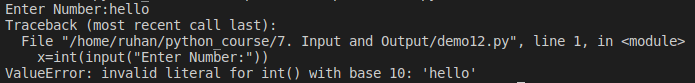Note: Exception Handling concept applicable for Runtime Errors but not for syntax errors.

##### Normal Flow of Program Execution in Python:

In a program, if all statements are executed as per the conditions successfully and if we get the output as expected then that flow is called the normal flow of the Program Execution. The below program will get executed successfully from start to end.

###### Program: Normal Flow (demo5.py)
```print('One')
print('Two')
print('Three')
print('Four')
print('Five')```
###### Output: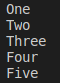##### Abnormal Flow of Program Execution in Python:

While executing statements in a program, if any error occurs at runtime, then immediately program flow gets terminated abnormally, without executing the other statements. This kind of termination is called an abnormal flow of execution. The following example terminated abnormally.

###### Program: Abnormal flow (demo6.py)
```print('One')
print('Two')
print(10/0)
print('Four')
print('Five')```
###### Output: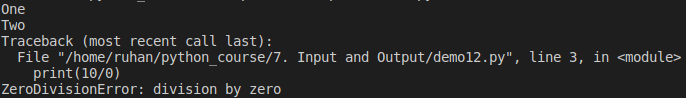Above program terminated in the middle of the execution where a run time error occurred. As discussed, if a runtime error occurs it won’t execute the remaining statements.

##### What is an Exception in Python?

An unwanted or unexpected event that disturbs the normal flow of the program is called an exception. Whenever an exception occurs, immediately the program will terminate abnormally. In order to get our program executed normally, we need to handle those exceptions on high priority.

##### Exception Handling in Python:

Exception handling does not mean repairing or correcting the exception. Instead, it is the process in which we define a way so that the program doesn’t terminate abnormally due to the exceptions.

##### Default Exception Handing in Python:

In python, for every exception type, a corresponding class is available and every exception is an object to its corresponding class. Whenever an exception occurs, Python Virtual Machine (PVM) will create the corresponding exception object and will check for handling code.

If handling code is not available, then the Python interpreter terminates the program abnormally and prints corresponding exception information to the console. The rest of the program won’t be executed.

##### Exception Hierarchy: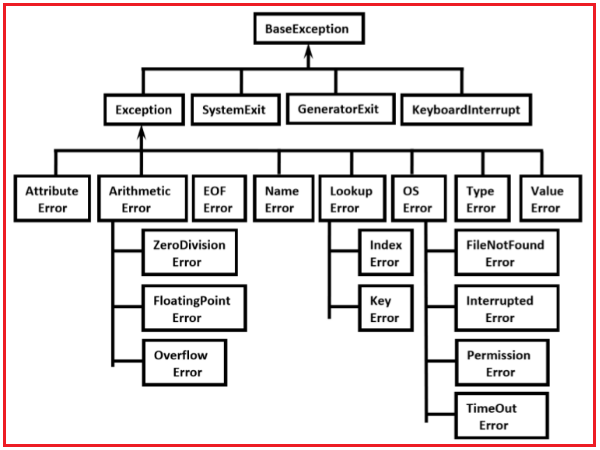Note: Every Exception in Python is a class. The BaseException class is the root class for all exception classes in the python exception hierarchy and all the exception classes are child classes of BaseException. The Programmers need to focus and understand clearly the Exception and child classes.

##### How to Handle Exceptions in Python?

Using Try-Except statements we can handle exceptions in python.

• Try block: try is a keyword in python. The code which may be expected to raise an exception should be written inside the try block.
• Except block: except is a keyword in python. The corresponding handling code for the exception, if occurred, needs to be written inside the except block.
###### Syntax: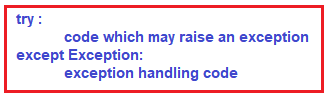##### How does it work?

If the code in the try block raises an exception, then only the execution flow goes to the except block for handling code. If there is no exception raised by the code in the try block, then the execution flow won’t go to the except block.

###### Program: Handling exception by using try and except (demo7.py)
```print('One')
print('Two')

try:
print(10/0)
except ZeroDivisionError:
print("Exception passed")
print('Four')
print('Five')```
###### Output: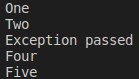Let’s see how the execution flow is, in different scenarios using ‘try except’

###### Case1:

In our program, if there is no exception, then should be normal flow with normal termination. In this case, the except block won’t execute.

###### Program: without Exception (demo8.py)
```print('One')
print('Two')

try:
print(10/2)
print("No Exception")
except ZeroDivisionError:
print("Exception passed")
print('Four')
print('Five')```
###### Output: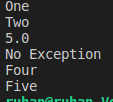##### Case 2:

In our program, if an exception occurs before the try block then the program terminates abnormally. Only the code inside the try block will be checked for the exception and its handling code. But, if there is any exception for the code before the try block, then abnormal termination

###### Program: program terminates abnormally (demo9.py)
```print(10/0)

try:
print(10/2)
print("No Exception")
except ZeroDivisionError:
print("Exception passed")
print('Four')
print('Five')```

Output: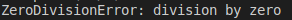##### Case 3:

If the code inside the try block raises an exception, then the execution flow goes to the except block. In the except block, we should have handled the corresponding exception that occurred in the try block, only then the except block will execute leading to normal termination.

###### Program: demo10.py
```print('One')
print('Two')
try:
print(10/0)
print("No Exception")
except ZeroDivisionError:
print("Exception passed")
print('Four')
print('Five')```
###### Output: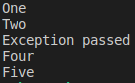##### Case 4:

If the code inside the try block raises an exception, then the execution flow goes to the except block. In the except block we should have handled for the exception other than what occurred in the try block, then the except block will not execute leading to abnormal termination.

###### Program: demo11.py
```try:
print(10/0)
print("No Exception")
except TypeError:
print("Exception passed")
print('Four')
print('Five')```

Output: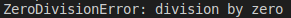##### Case 5:

If there are 3 lines of code inside the try block and an exception is raised when executing the first line, then the execution flow goes to the except block without executing the remaining two lines.

###### Program: demo12.py
```try:
print(10/0)
print("line 2")
print("line 3")
print("No Exception")
except ZeroDivisionError:
print("Exception passed")
print('Four')
print('Five')```
###### Output: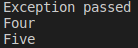#### Case 6:

If an exception is raised inside the try block, the execution goes to the except block. If the code inside the except block also raises an exception then it leads to abnormal termination

###### Program: demo13.py
```try:
print(10/0)
print("No Exception")
except ZeroDivisionError:
print(10/0)
print('Four')
print('Five')```
###### Output: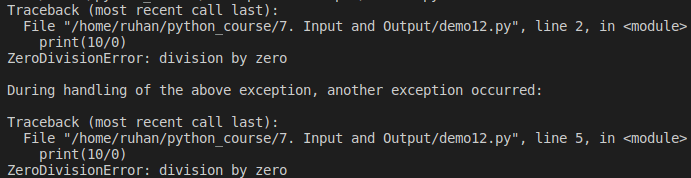##### Case 7:

If an exception is raised inside the try block, the execution goes to the except block. After the execution of the code inside the except block, the execution comes out of it and continues with the other statements that follow. If any exceptions occur, when executing those following statements, then it leads to abnormal termination.

###### Program: demo14.py
```try:
print(10/0)
except ZeroDivisionError:
print("Exception passed")
print(10/0)
print('Four')
print('Five')```
###### Output: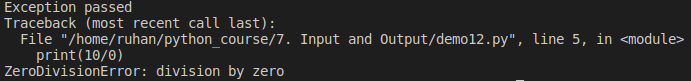##### Printing Exception Information in Python:

In the above examples, in the exception handling code, we are printing our custom message like “Exception passed” etc. Rather than that, we can also print exception information by creating a reference to the exception.

###### Program: Printing exception information (demo15.py)
```try:
print(10/0)
except ZeroDivisionError as z:
print("Exception information:", z)```

Output: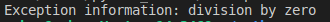##### try with multiple except Blocks in python:

In python, try with multiple except blocks are allowed. Sometimes, there may be the possibility that a piece of code can raise different exceptions in different cases. In such cases, in order to handle all the exceptions, we can use multiple except blocks. So, for every exception type a separate except block we have to write.

###### Syntax: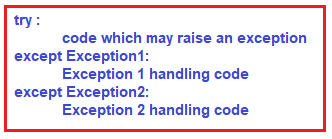###### Program: try with Multiple Except Blocks (demo16.py)
```try:
x=int(input("Enter First Number: "))
y=int(input("Enter Second Number: "))
print(x/y)
except ZeroDivisionError:
print("Can't Divide with Zero")
except ValueError:
###### Output1: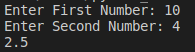###### Output2: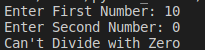###### Output3: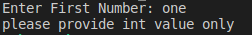###### Output4: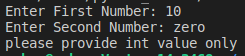##### ONE Except BLOCK – MULTIPLE EXCEPTIONS

Rather than writing different except blocks for handling different exceptions, we can handle all of them in one except block. The only thing is we will not have the flexibility of customizing the message for each exception. Rather, we can take the reference to the exception and print its information as shown in one of the examples above.

###### Syntax: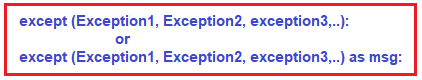Parenthesis are mandatory, and this group of exceptions is internally considered as a tuple.

###### Program: single except block handling multiple exceptions (demo17.py)
```try:
x=int(input("Enter First Number: "))
y=int(input("Enter Second Number: "))
print(x/y)
except (ZeroDivisionError,ValueError) as e:
print("Please Provide valid numbers only and problem is: ", e)```
###### Output1: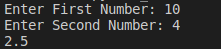###### Output2: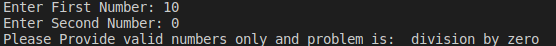###### Output3: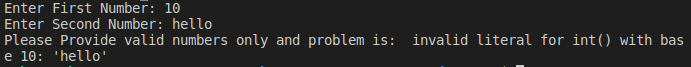###### Output4: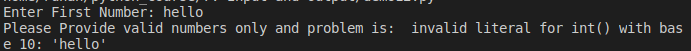##### Default Except Block in Python:

We can use default except block to handle any type of exceptions. It’s not required to mention any exception type for the default block. Whenever we don’t have any idea of what expectation the code could raise, then we can go for default except block.

We know that we can have multiple except blocks for a single try block. If the default except block is one among those multiple ones, then it should be at last, else we get SyntaxError.

###### Program: Default except block (demo18.py)
```try:
x=int(input("Enter First Number: "))
y=int(input("Enter Second Number: "))
print(x/y)
except ZeroDivisionError:
print("ZeroDivisionError: Can't divide with zero")
except:
print("Default Except: Please provide valid input only")```
###### Output1: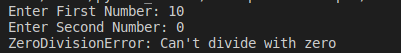###### Output2: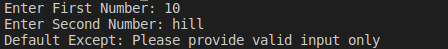###### Program: Default block (demo19.py)
```try:
x=int(input("Enter First Number: "))
y=int(input("Enter Second Number: "))
print(x/y)
except:
print("Default Except: Please provide valid input only")
except ZeroDivisionError:
print("ZeroDivisionError: Can't divide with zero")```

Output: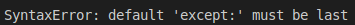1.2.